# Deep tensor networks with the tensor-network attention layerBojan Žunkovič

Tensor networks are ubiquitous in the field of many-body quantum physics. Since 2014 they have increasingly been applied also in machine learning. So far, two main applications of tensor networks in machine learning are the compression of large weight layers and a new type of multilinear model. Tensor networks are also a stepping stone toward applicable quantum machine learning. However, we typically use tensor networks as single-layer linear models in exponentially large Hilbert space. In this post, I will describe a non-linear tensor-network layer, which we can use to build deep tensor networks. I will discuss applications of deep tensor networks to specific datasets and tasks in the following posts.

This post is related to two papers:

## Architecture

A standard tensor network model consists of two parts. The first part is the embedding, which transforms the raw input (categorical or numerical) into vectors of size $d$. The second part is an exponentially large linear model with weights given/compressed in a tensor-network form. Although the first embedding part is important, I will focus on the second part. We will assume that we have an embedding layer that transforms the input of length $N$ into a Hilbert space vector of size $d^N$ via a local embedding input features $x_i$, i.e. $x_j\rightarrow \phi(x_j)$.We then write the embedding of a single input vector as a tensor product of the local embeddings, $\Phi(x)=\phi(x_1)\otimes\phi(x_2)\ldots\phi(x_N)$.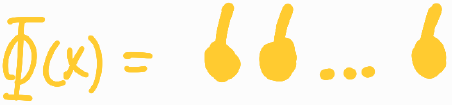### Tensor network attention

In the following, we will transform the embedded inputs with the tensor-network (TN) attention layer determined by an attention tensor $A\in R^{D\times D\times d}$ and a transformation tensor $B\in R^{D\times D\times d \times d}$.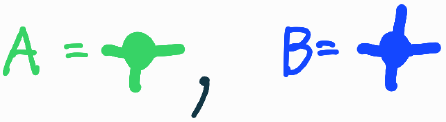We will keep the tensors $A$ and $B$ constant for any input position. The generalization of uniform to non-uniform TN attention is straightforward.

First, we construct matrices $\mathcal{A}(j)$ by contracting the attention tensor $A$ with the local embedding vectors $\phi(x_j)$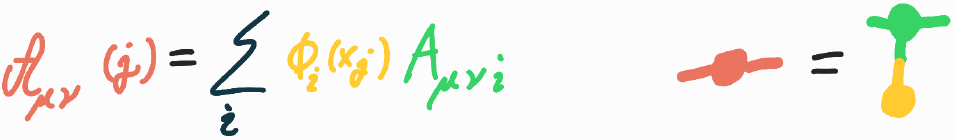Then, we use the matrices $\mathcal{A}(j)$ to construct the left and right context matrices $H^{\rm L,R}(j)$,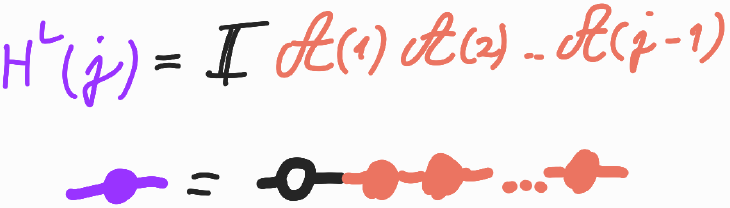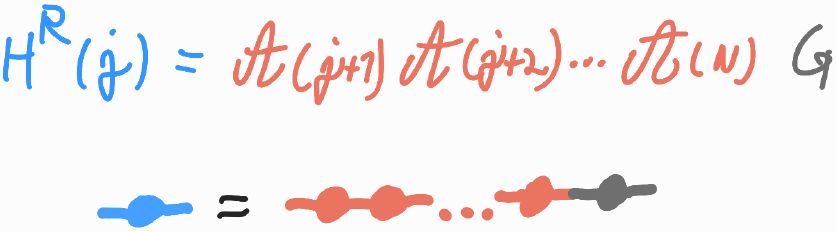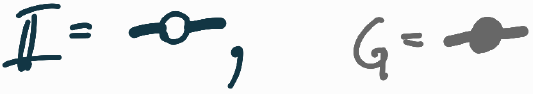The matrix $G$ determines the boundary conditions. In the case of closed/cyclic boundary conditions, we use $G=1_{D}$. In the case of open boundary conditions, we use $G=v^{\rm L}\otimes v^{\rm R}$, where the boundary vectors $v^{\rm L,R}\in R^d$ are determined as left and right eigenvectors of the matrix $A_0$ corresponding to the largest eigenvalue.

In the next step we calculate tensor $\mathcal{B}(j)$ by contracting the embedding at position $j$, i.e. $\phi(x_j)$ with the transformation tensor $B$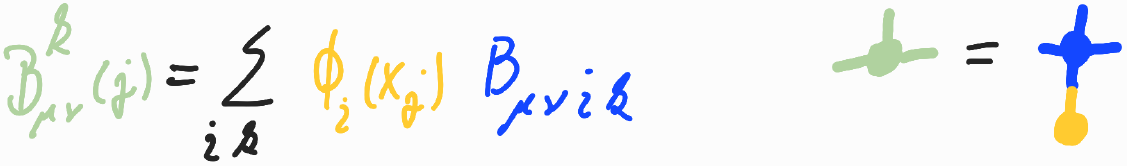where $B_{j,k}$ denotes the matrix with elements $[B_{j,k}]_{\mu,\nu}=B_{\mu,\nu,j,k}$. Finally, we contract the context matrices with the tensor $\mathcal{B}(j)$ to obtain the transformation of the local embedding $\phi(x_j)$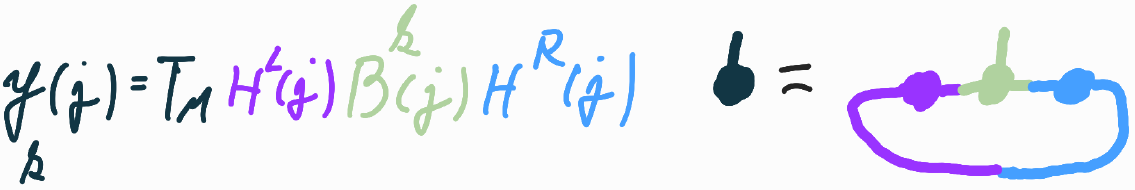Optionally, we can add a bias and a final non-linearity. In some variants of the TN attention, we use four-dimensional attention tensors $A\in R^{D\times D\times d\times d}$ and construct $\mathcal{A}(i)$ by contracting $A$ with two copies of the local embeddings.

The entire embedding is then transformed by the TN attention as $\Phi(x)\rightarrow y(1)\otimes y(2)\otimes\ldots\otimes y(N)$### Short diagrammatic overview

Finally, let us summarize the TN attention layer in a diagrammatic notation by using the definitions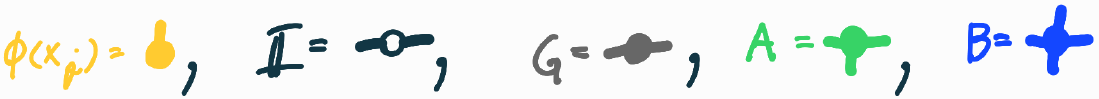We summarize the action of a TN attention on an embedding $\phi(j)$ in three steps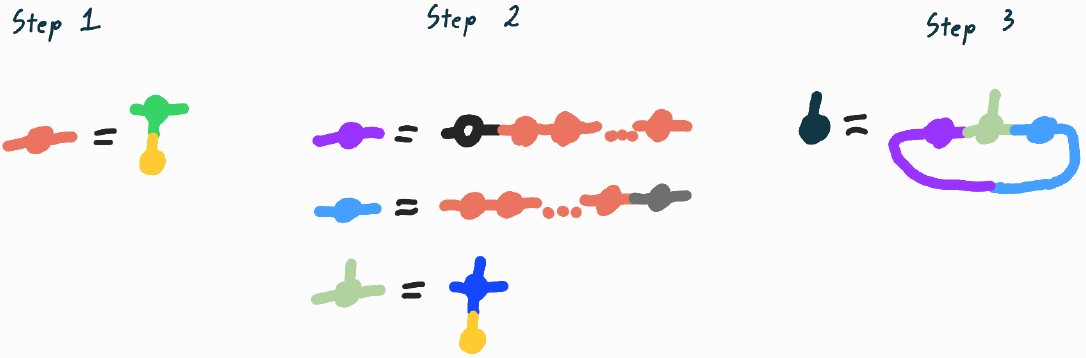### Local weight matrix

We can also represent the TN attention layer as a local weight matrix $W(j)$ acting on the embedding $\phi(x_j)$. The diagrammatic definition of the local weight matrix is shwon in next figure.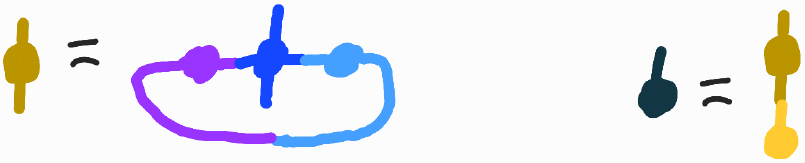### Generalised attention tensor

We can add the attention tensors one more dimension, i.e., $A\in R^{D\times D\times d\times d}$. In this case, we calculate the matrices $\mathcal{A}(j)$ by contracting the attention tensors $A$ with two copies of the embedding vectors.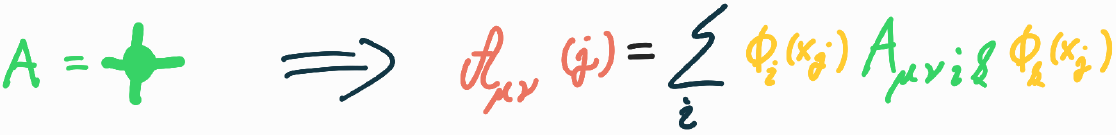Alternatively, we could introduce a new local embedding map as $\tilde{\phi}(x_j)=\phi(x_j)\otimes \phi(x_j)$ and keep the definition of $A$. This transformation would lead to the same left and right context matrices but a slightly more general weight matrix $W$.

## Deep tensor networks

The main benefit of the TN attention layer is that the bond dimension of the input matrix product state (the embedding vectors) does not increase with the layer application. Therefore, we can apply the TN attention layer repeatedly. We call the resulting model a deep tensor network. The final architecture is shown in the figure below and consists of an embedding layer followed by the TN attention layers. We also add a skip connection and normalization, which we can turn off. The final layer depends on the task. In the classification case, we use the MPS layer described in a previous post on MPS baselines. In the sequence prediction case, the final layer is a decoder layer. The decoder is a simple inverse operation of the embedding layer. We will discuss the sequence prediction case in one of the following posts on grokking.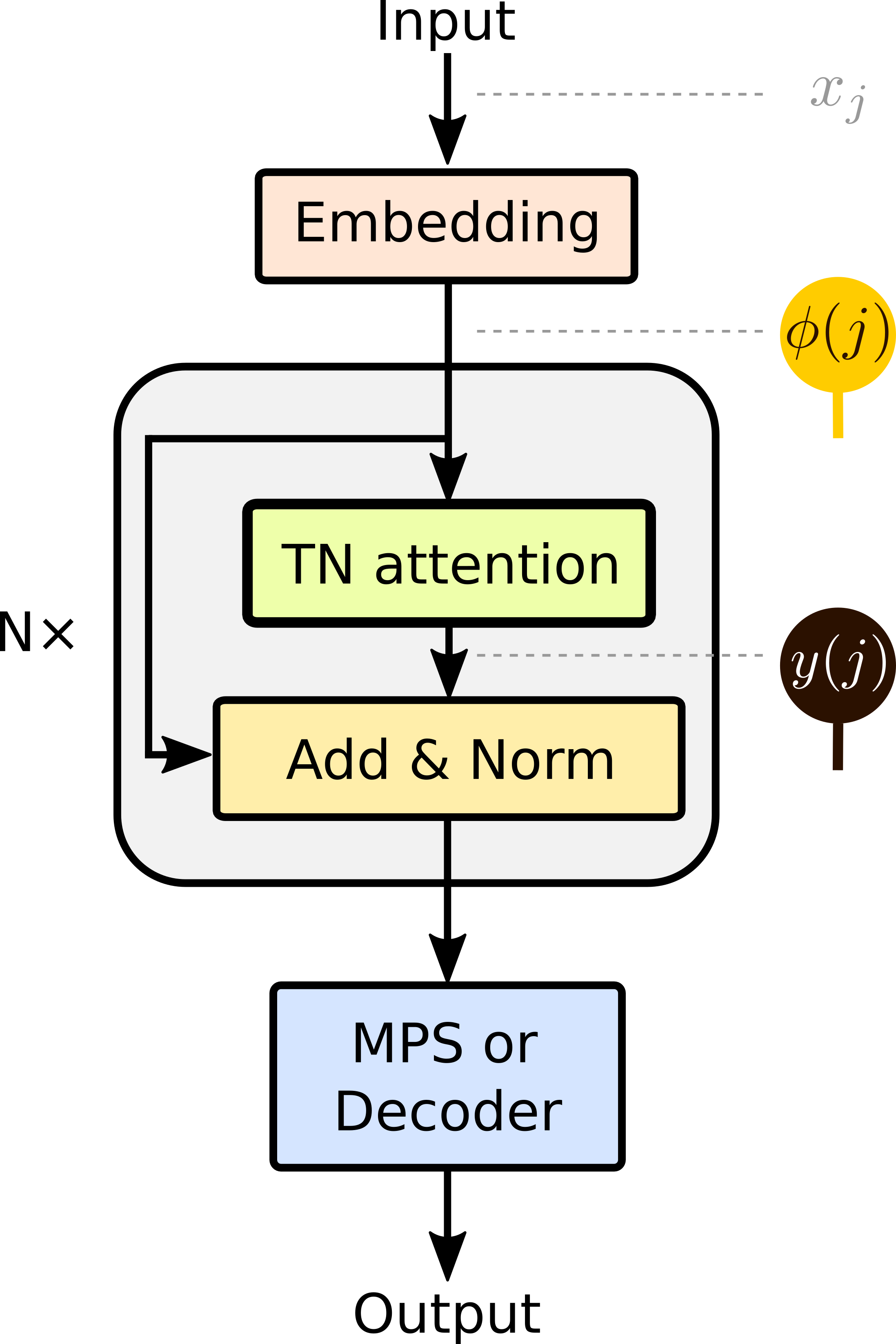## TN attention as generalized linear-dot attention

I will not explain how we can rewrite a linear dot-attention as a TN attention layer with the bond dimension $D = d^2+1$. In particular, we show that a slight modification of the proposed TN attention layer implements the linear dot-attention transformation $y_j=(q_j\cdot k_l)q_l,$ where $q_j=W^{\rm Q}\phi(j)$, and $k_j=W^{\rm K}\phi(j)$. For simplicity we removed the factor $\frac{1}{\sqrt{d}}$ and used a trivial transformation of values ($v_j=\phi(j)$). We obtain a more general transformation by applying the linear transformation $W^{\rm V}(W^{\rm Q})^{-1}$ on the transformed values $y_j$. Additionally, we assume that the embedding vectors are $L_2$ normalized.

The embedding tensor can be interpreted as an $N$-fold tensor product of embedding vectors $\Phi=\phi(1)\otimes\phi(2)\otimes\ldots\otimes\phi(N)$. We consider the action of the TN attention on the vector $\Phi$. First, we decompose TN attention tensors $A\in R^{D\times D\times d\times d}$ into three components

$A^{t,s}_{a,a'}(j)=\sum_{t',s'=1}^{d}W^{\rm Q}_{t',t}\tilde{A}^{t',s'}_{a,a'}(j)W^{\rm K}_{s',s}$

The matrices $W^{\rm Q}$ and $W^{\rm K}$ transform the local vectors $\phi(j)$ to queries and keys. The remaining TN attention implements a permutation operator

${\mathrm Tr}\left(\tilde{G} \tilde{A}^{t_1,s_1}\tilde{A}^{t_2,s_2}\ldots\tilde{A}^{t_N,s_N}\right) = \sum_{i

where

$P_{i,j}\phi(1)\otimes\phi(2)\otimes\ldots\phi(i)\otimes\ldots\phi(j)\otimes\ldots\phi(N) = \phi(1)\otimes\phi(2)\otimes\ldots\phi(j)\otimes\ldots\phi(i)\otimes\ldots\phi(N)$

The local weight matrix $W(j)$, without the normalization of the left and right context matrices, is then given by

$W(j) = \sum_{i,l\neq j}(q_i\cdot k_l)(k_i\cdot q_l) 1_d + \sum_{i\neq j} q_i k_i^T.$

The final transformation of the embedding $\phi(j)$ with the described TN attention layer is then

$\phi(j)\rightarrow c q_j + \sum_{i\neq j}q_i (k_i\cdot q_j),$

where $c=\sum_{i,l\neq j}(q_i\cdot k_l)(k_i\cdot q_l)$. We can correct the difference between the linear attention and the TN attention result by a simple local residual connection. The final difference is that we normalize the left and the right context matrices. This normalization translates to rescaling the final output and has no effect if we normalize the output after each application of the attention mechanism.

Finally, we provide the TN attention tensors $\tilde{A}$ that implement the permutation transformation

$\tilde{A}^{t,s}_{1,dt+s+1}=1,\quad t,s=1,2\ldots d,$

$\tilde{A}^{s,t}_{dt+s+1,1}=1,\quad t,s=1,2\ldots d,$

$\tilde{A}^{s,s}_{a,a}=1,\quad s=1,2\ldots d,\quad a=2,3\ldots d^2+1,$

$\tilde{G}_{1,1}=1.$

The remaining elements of the tensors $\tilde{A}$ and $\tilde{G}$ are zero.

As we have just seen, the TN attention layer can implement (though in an inefficient way) linear dot attention. In addition, the TN attention can model higher-order correlations/interactions between embeddings and explicitly incorporates the embedding position. Therefore, we interpret the tensor-network attention as a generalized linear dot attention mechanism. On the other hand, we can not straightforwardly introduce nonlinearities to the presented formalism.

I will discuss the application of the TN attention layer/formalism to the image classification problem and grokking in the following posts.